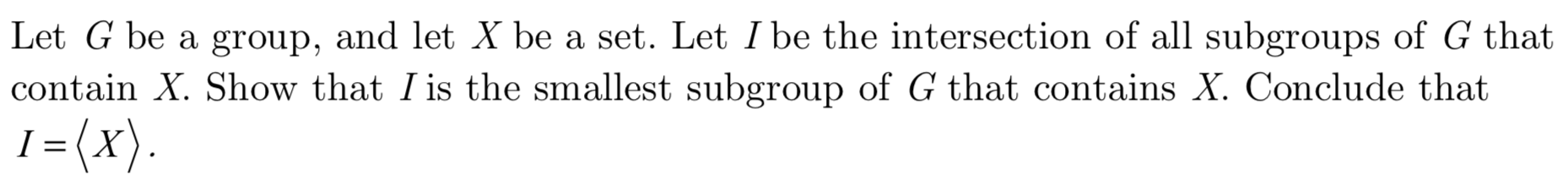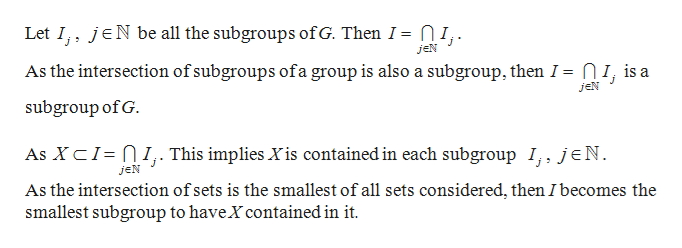# Let G be a group, and let X be a set. Let I be the intersection of all subgroups of G thatcontain X. Show that I is the smallest subgroup of G that contains X. Conclude that1= (x)I

Question
6 viewshelp_outlineImage TranscriptioncloseLet G be a group, and let X be a set. Let I be the intersection of all subgroups of G that contain X. Show that I is the smallest subgroup of G that contains X. Conclude that 1= (x) I fullscreen
check_circle

Step 1

Let G be a group and X be a set in G. Suppose I is the intersection of all subgroups of G that contains X.

To prove:

Step 2

Proof:

...help_outlineImage TranscriptioncloseLet I jN be all the subgroups ofG. Then I = NI,. J jeN As the intersection of subgroups ofa group is also a subgroup, then I = nI, is a jeN subgroup of G. As XCI nI This implies X is contained in each subgroup I, jeN. jeN As the intersection ofsets is the smallest of all sets considered, then I becomes the smallest subgroup to have Xcontained in it fullscreen

### Want to see the full answer?

See Solution

#### Want to see this answer and more?

Solutions are written by subject experts who are available 24/7. Questions are typically answered within 1 hour.*

See Solution
*Response times may vary by subject and question.
Tagged in

### Math